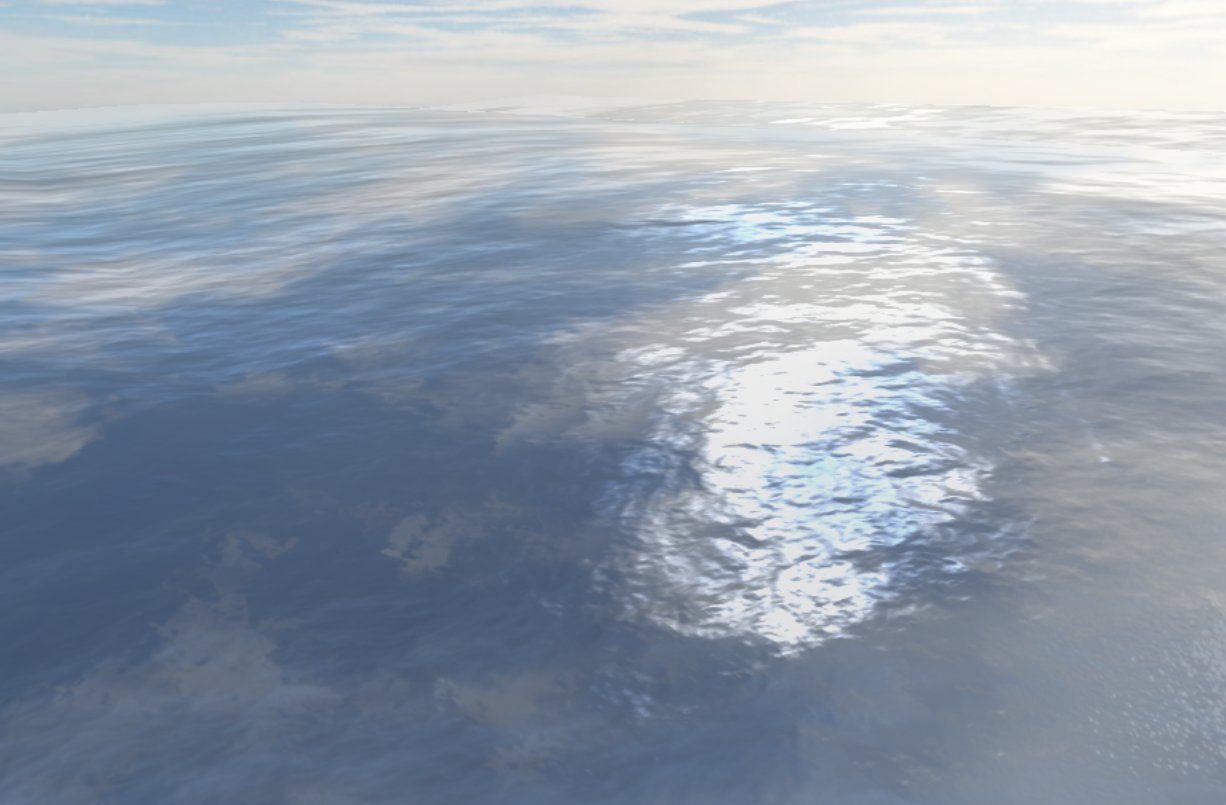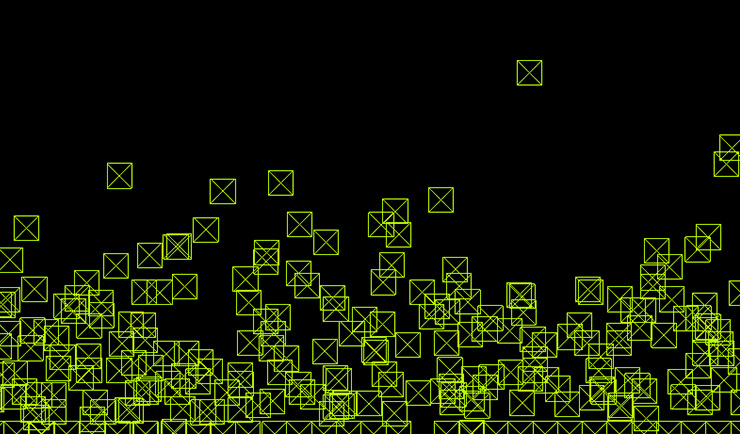Water surface simulation summing simple sine functions in ThreeJS using vertex shaders

Finished at April 1, 2017A height map is calculated dynamically summing simple sine functions

This is a simple surface ocean water simulation based on a sum of sine functions approach for calculate a height map that fits as a normal extrusion coefficient in a plane mesh and that dynamically calculates water surface orientation from GPU in a vertex shader. Visualization characteristics are provided by Jeremy Bouny water projects made in ThreeJS @jbouny/ocean . This Project could be considered a basic implementation of GPU gems chapter 1, named Effective Water Simulation from Physical Models By Mark Finch and Cyan Worlds.

2D SPH Water simulation in ThreeJS from CPU

Finished at May 25, 2017Every water particle is represented as a green box of width and heigh h

This is a basic water body simulation based on the Smoothed Particle Hydrodynamic (SPH) method with leapfrog integrator for simulate water fluids in 2D from CPU using JavaScript and ThreeJS. The code was originally written in C by the Computer Science professor David Bindel at Cornell University as a parallel computing class exercise although this is a serial implementation (no parallel computing is involved).
The port from C to JavaScript was made in collaboration with @jorovipe97.

OpenGL and WebGL course

My developed material for an OpenGL/WebGL semminar workshop.

01 Content | The content of the course.

Where is OpenGL / WebGL used?

02 introduction | What is OpenGL?

The inner workings of computer graphics.

03 Windowing | OpenGL Window

Creating the window and context for our application.

04 Modern pipeline | Understanding the pipeline

Understanding the order in which GPU things happen.

06 Transformations | Coordinate systems

Vector, matrices and GLM.

07 Lighting | Lighting fundamentals

Standard phong model.

08 Lighting II | Diffuse and Specular maps

Improving quality with textures.

09 Effects | Frame Buffer Objects

Creating simple postproduction effects.

10 WebGL Introduction | Tools and languages

Real tools used in production environments.

11 ThreeJS | Using WebGL libraries

Using Three JS to fast up the work.

Simulation for Interactive Systems course

My developed material for the Simulation for Interactive Systems course.

01 Content | The content of the course

Where to go using some maths?

02 Vectors | What are vectors?

Imagining the vectors.

03 Motion | Vectors and motion

Displacement, velocity and acceleration.

04 Forces | Modeling forces

Understanding the physic forces.

05 Forces II | Modeling forces

Translating formulas to code.

06 Trigonometry | Trigonometric function and its inverses

Interpreting fundamental trigonometric functions.

07 Coordinate spaces | Matrices

Understanding matrices in Computer Graphics.

08 Oscillations | Trigonometry and physics

Simple applications of trigonometric functions on physics.

-